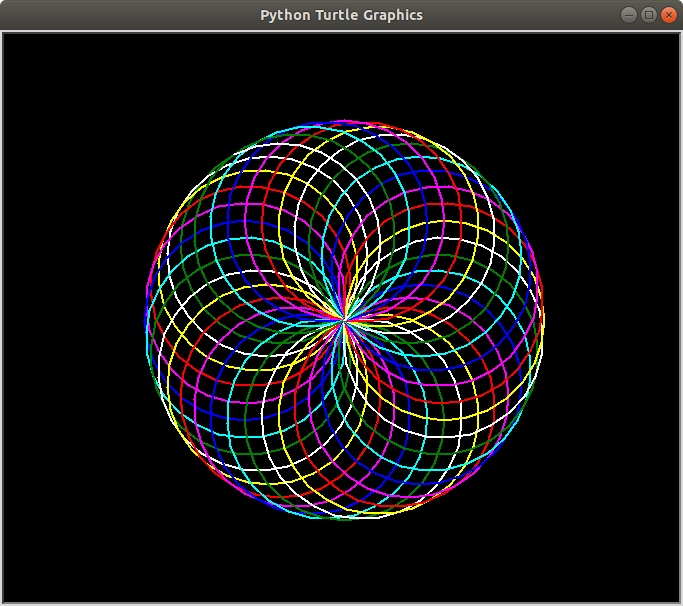Open in App
Not now

# Print a Spirograph using turtle in Python

• Difficulty Level : Basic
• Last Updated : 11 Sep, 2021

Pre-requisites: Turtle Programming in Python

A spirograph is a very interesting geometrical figure which is often symmetrical to both the axes. It produces mathematical roulette curves of the variety technically known as hypotrochoids and epitrochoids. Here, we’ve used a range of colors to draw circles, you can use your combination as per your color choice.

Below is the implementation.

## Python3

 `# Import the turtle library for``# drawing the required curve``import` `turtle as tt` `# Set the background color as black,``# pensize as 2 and speed of drawing``# curve as 10(relative)``tt.bgcolor(``'black'``)``tt.pensize(``2``)``tt.speed(``10``)` `# Iterate six times in total``for` `i ``in` `range``(``6``):``  ` `      ``# Choose your color combination``    ``for` `color ``in` `(``'red'``, ``'magenta'``, ``'blue'``,``                  ``'cyan'``, ``'green'``, ``'white'``,``                  ``'yellow'``):``        ``tt.color(color)``        ` `        ``# Draw a circle of chosen size, 100 here``        ``tt.circle(``100``)``        ` `        ``# Move 10 pixels left to draw another circle``        ``tt.left(``10``)``    ` `    ``# Hide the cursor(or turtle) which drew the circle``    ``tt.hideturtle()`

Output:My Personal Notes arrow_drop_up